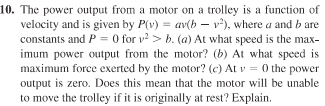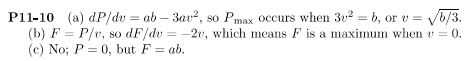# Power from trolley motor

walking
Homework Statement:
For part (c) author says F=ab when v=0. I don't see how since Fv=av(b-v^2) doesn't necessarily mean F=ab at v=0. In fact F can take any value?
Relevant Equations:
Fv=av(b-v^2)Author solution:Homework Helper
Gold Member
2022 Award
Homework Statement:: For part (c) author says F=ab when v=0. I don't see how since Fv=av(b-v^2) doesn't necessarily mean F=ab at v=0. In fact F can take any value?
Relevant Equations:: Fv=av(b-v^2)

View attachment 279836

Author solution:

View attachment 279837
From a strictly mathematical perspective you are right, but it is reasonable to assume that F is a continuous function of v, so you can take the limit as v tends to zero.

walking
From a strictly mathematical perspective you are right, but it is reasonable to assume that F is a continuous function of v, so you can take the limit as v tends to zero.
Does this only work for continuous functions? If so why?

I do not understand how an author got that answer. That result, if possible at all, is possible only in one way when you make such equation: $$Fv = av(b-v^2)$$ Then you divide by v and get this formula $$F= \frac {av(b-v^2)} {v}.$$ You can, obviously simplify it and you get your $$F = a(b-v^2)= ab, v=0$$ But if the object is not moving the power output is zero (also written in a task). But I'm wondering where the force equation comes from. And if this force exists what is this force at all, what it exerted to, and what it is equilibrated by? (the case when object does not move)

IMHO, this questions is smth like: you sit on a chair, your velocity equals zero, does it mean you cannot stand up? If there is a deeper idea in it, tell me please.

Last edited:
•Lnewqban
Homework Helper
Gold Member
2022 Award
Does this only work for continuous functions? If so why?
You can, obviously simplify it and you get
We are told P(v), not F(v). If we do not assume F is continuous then it could be
0 when v=0
a(b-v2) otherwise.
If there is a deeper idea in it, tell me please.
How can you start an object moving from rest if the power is given by Fv and v is zero?
We can maybe explain it in terms of limits: over any nonzero time interval dt there is work done and a nonzero average power. If we say that power is not a fundamental concept and is only defined in terms of an average over a time interval then the problem goes away.
Or perhaps, in the real world, we should be considering the Planck length, etc.?

Last edited:
•Lnewqban
Homework Helper
Gold Member
... But I'm wondering where the force equation comes from. And if this force exists what is this force at all, what it exerted to, and what it is equilibrated by? (the case when object does not move)
It seems to me that the force of reference is the result of the initial torque from the electric motor, applied to the wheel(s), just before the trolley starts moving forward.
That would be a tangential force at the contact point of the wheel with the rolling surface, transferring momentum to the accelerating mass of the whole trolley.
Just like the instantaneous situation of a pendulum at maximum swing position, there is a force and an acceleration, but its velocity is zero.